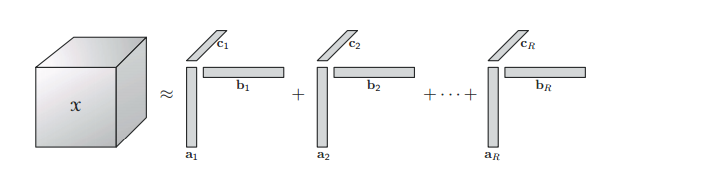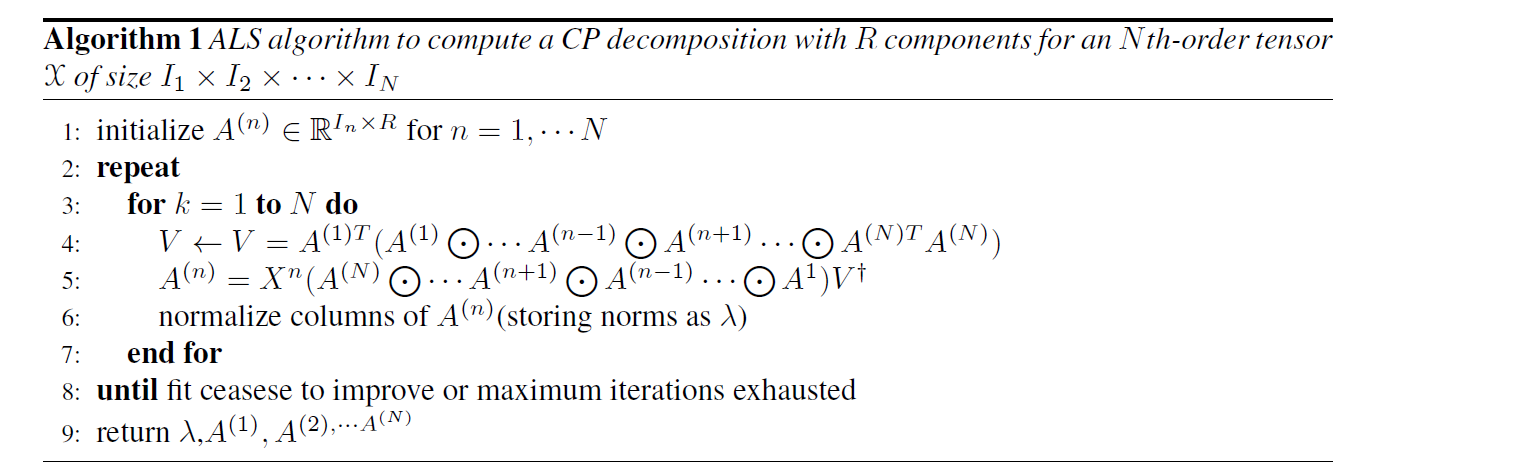## CP分解(Canonical Polyadic Decomposition)

1927年Hitchcock提出了CP 分解。CP 分解将一个$N$阶的张量$\mathcal{X}\in {\mathbb{R}^{{I_1}{\mathbf{ \times }}{I_2}{\mathbf{ \times }} \cdots {\mathbf{ \times }}{I_N}}}$分解为$R$个秩为1的张量和的形式即: \begin{equation} \mathcal{X}=\sum \limits_{{r} = 1}^{{R}} \lambda_{r} a_{r}^{(1)}\circ a_{r}^{(2)}\circ \cdots a_{r}^{(N) }
\end{equation} 通常情况下$a_{r}^{(n)}$是一个单位向量。定义$A^{(n)}=[a_{1}^{n} \quad a_{2}^{n} \quad \cdots \quad a_{R}^{n}]$,$D=diag(\lambda)$ 那么上面的公式可以写为: \begin{equation} \mathcal{X}=D\times_{1}A^{(1)}\times_{2}A^{(2)}\cdots\times_{N}A^{(N)} \end{equation} 矩阵的表达形式即为： \begin{equation} X_{(n)}=A^{n}D(A^{(N)} \bigodot \cdots A^{(n+1)} \bigodot A^{(n-1)}\cdots \bigodot A^{1})^T \label{eq:cpmatix} \end{equation} 特殊的时候当张量$\mathcal{X}$的阶数为3的时候,它的分解的形式下图所示。## CP的求解

CP分解的求解首先要确定分解的秩1张量的个数，正如前面介绍的由于张量的秩Rank-n 近似无法渐进地得到。通常我们通过迭代的方法对$R$从1开始遍历直到找到一个合适的解。当数据无噪声时，重构误差为0所对应的解即为CP分解的解，当数据有噪声的情况下，可以通过CORCONDIA算法估计$R$。当分解的秩1张量的个数确定下来之后，可以通过交替最小二乘方法对CP分解进行进行求解。下面我们以三阶张量为例对ALS 算法进行阐述。

\begin{equation} \min \limits_{\mathcal{\hat{X}}} \| \mathcal{X}- \hat{\mathcal{X}\|},\quad \hat{\mathcal{X}}=\sum \limits_{r=1}^{R}\lambda_{r} a_{r} \circ b_{r} \circ c_{r}=\left[\kern-0.15em\left[ {\lambda ; A,B,C} \right]\kern-0.15em\right] \label{eq:cpobj} \end{equation} ALS算法首先固定$B$,$C$去求解$A$，接着固定$A$和$C$去求解$B$,然后固定$B$ 和$C$去求解$A$,这样不断地重复迭代直到达到收敛条件。固定矩阵$B$和$C$,可以得到上面的式子在mode-1矩阵展开的时候形式为 \begin{equation} \min \limits_{\hat{A}}=\|X_{(1)}-\hat{A}(C \odot B)^{T} \|_{F}\label{eq:cpmode1} \end{equation} 其中$\hat{A}=A.diag(\lambda)$,那么上述式子的最优解为 \begin{equation} \hat{A}=X_{(1)}[(C \odot B)^{T}]^{\dagger}\label{eq:cpmode1solution} \end{equation} 为了防止数值计算的病态问题，通常把$\hat{A}$的每一列单位化，即 $\lambda_{r}=|\hat{a_{r}}|,\quad a_{r}=\hat{a_{r}}/\lambda_{r}$ 将上述式子推广到高阶形式可以得到 \begin{equation} \begin{split} &A^{(n)}=X^{n}(A^{(N)} \bigodot \cdots A^{(n+1)} \bigodot A^{(n-1)}\cdots \bigodot A^{1} )V^{\dagger} \
&where \quad V=A^{(1)T}(A^{(1)} \bigodot \cdots A^{(n-1)} \bigodot A^{(n+1)}\cdots \bigodot A^{(N)T}A^{(N)} ) \end{split} \end{equation} 对于一个$N$阶的张量$X$它的ALS求解的步骤如下图所示。它假设分解出Rank-1 张量的个数$R$是事先知道的,对于各个因子的初始化，可以采用随机初始化也可以通过下面的式子进行初始化。 \begin{equation} A^{(n)}=R \quad \text{ leading left singular vectors of} X_{(n)} \text{for} n=1,\cdots N \end{equation}## CP分解应用

CP分解已经在信号处理,视频处理,语音处理,计算机视觉、机器学习等领域得到了广泛的应用 下面，以去噪为例,对CP分解在高光谱图像处理中的应用进行阐述。